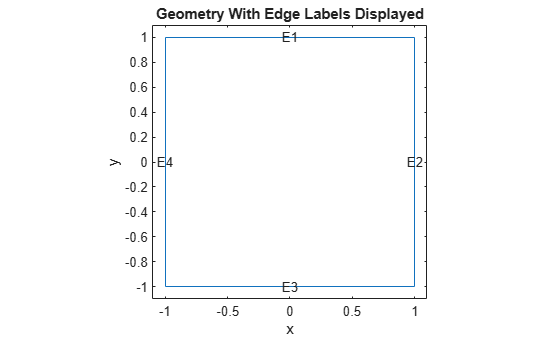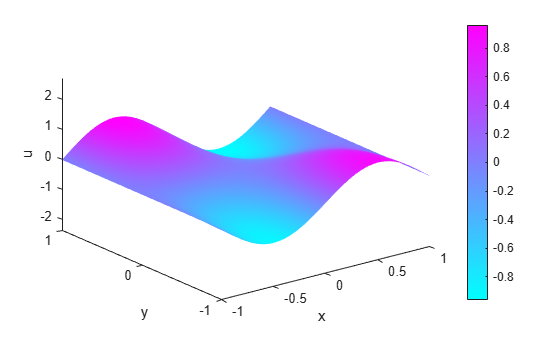Documentation

### This is machine translation

Mouseover text to see original. Click the button below to return to the English version of the page.

To view all translated materials including this page, select Country from the country navigator on the bottom of this page.

## Wave Equation on Square Domain

This example shows how to solve the wave equation using the `solvepde` function.

### Problem Definition

The standard second-order wave equation is

``

To express this in toolbox form, note that the `solvepde` function solves problems of the form

``

So the standard wave equation has coefficients,,, and.

```c = 1; a = 0; f = 0; m = 1; ```

### Geometry

Solve the problem on a square domain. The `squareg` function describes this geometry. Create a `model` object and include the geometry. Plot the geometry and view the edge labels.

```numberOfPDE = 1; model = createpde(numberOfPDE); geometryFromEdges(model,@squareg); pdegplot(model,'EdgeLabels','on'); ylim([-1.1 1.1]); axis equal title 'Geometry With Edge Labels Displayed'; xlabel x ylabel y ```### Specify PDE Coefficients

```specifyCoefficients(model,'m',m,'d',0,'c',c,'a',a,'f',f); ```

### Boundary Conditions

Set zero Dirichlet boundary conditions on the left (edge 4) and right (edge 2) and zero Neumann boundary conditions on the top (edge 1) and bottom (edge 3).

```applyBoundaryCondition(model,'dirichlet','Edge',[2,4],'u',0); applyBoundaryCondition(model,'neumann','Edge',([1 3]),'g',0); ```

### Generate Mesh

Create and view a finite element mesh for the problem.

```generateMesh(model); figure pdemesh(model); ylim([-1.1 1.1]); axis equal xlabel x ylabel y ```### Create Initial Conditions

The initial conditions:

•.

•.

This choice avoids putting energy into the higher vibration modes and permits a reasonable time step size.

```u0 = @(location) atan(cos(pi/2*location.x)); ut0 = @(location) 3*sin(pi*location.x).*exp(sin(pi/2*location.y)); setInitialConditions(model,u0,ut0); ```

### Define Solution Times

Find the solution at 31 equally-spaced points in time from 0 to 5.

```n = 31; tlist = linspace(0,5,n); ```

### Calculate the Solution

Set the `SolverOptions.ReportStatistics` of `model` to `'on'`.

```model.SolverOptions.ReportStatistics ='on'; result = solvepde(model,tlist); u = result.NodalSolution; ```
```456 successful steps 37 failed attempts 988 function evaluations 1 partial derivatives 112 LU decompositions 987 solutions of linear systems ```

### Animate the Solution

Plot the solution for all times. Keep a fixed vertical scale by first calculating the maximum and minimum values of `u` over all times, and scale all plots to use those-axis limits.

```figure umax = max(max(u)); umin = min(min(u)); for i = 1:n pdeplot(model,'XYData',u(:,i),'ZData',u(:,i),'ZStyle','continuous',... 'Mesh','off','XYGrid','on','ColorBar','off'); axis([-1 1 -1 1 umin umax]); caxis([umin umax]); xlabel x ylabel y zlabel u M(i) = getframe; end ```To play the animation, use the `movie(M)` command.

##### SupportGet trial now